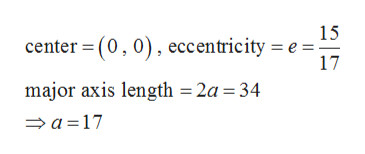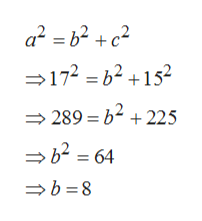# For Exercise, write the standard form of an equation of the ellipse subject to the following conditions.Center: (0, 0); Eccentricity: 15/17; Major axis vertical of length 34 units

Question
1 views

For Exercise, write the standard form of an equation of the ellipse subject to the following conditions.

Center: (0, 0); Eccentricity: 15/17; Major axis vertical of length 34 units

check_circle

Step 1

Given,help_outlineImage Transcriptionclose15 center = (0,0), eccentricity = e = 17 major axis length = 2a = 34 > a =17 fullscreen
Step 2

Now

Step 3

Now using the relation between semi major axis(a), semi minor axis(b) and t...help_outlineImage Transcriptionclosea² = b² +c² =172 = 62 +152 = 289 = b2 + 225 → 62 = 64 =b =8 fullscreen

### Want to see the full answer?

See Solution

#### Want to see this answer and more?

Solutions are written by subject experts who are available 24/7. Questions are typically answered within 1 hour.*

See Solution
*Response times may vary by subject and question.
Tagged in

### Algebra